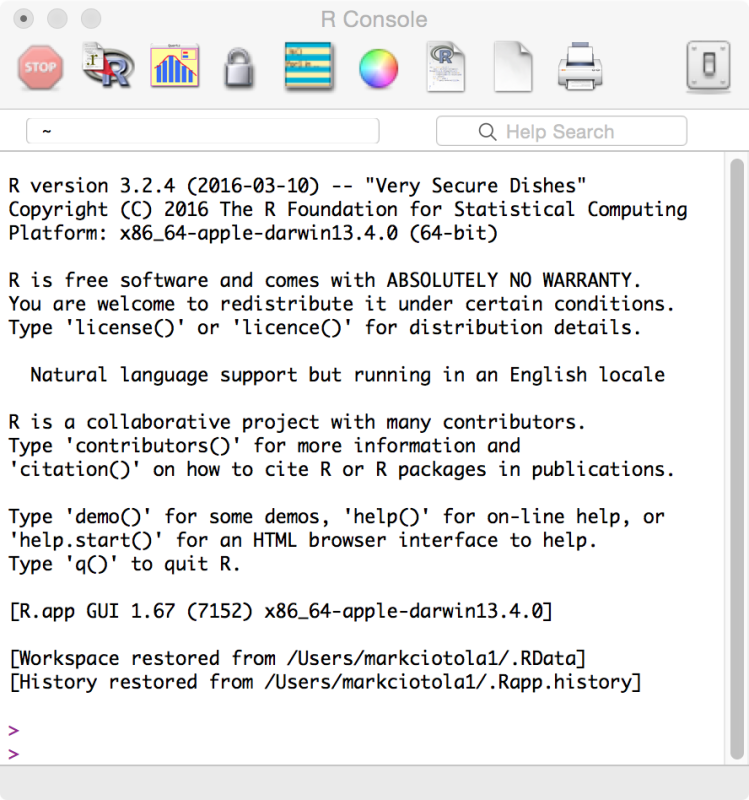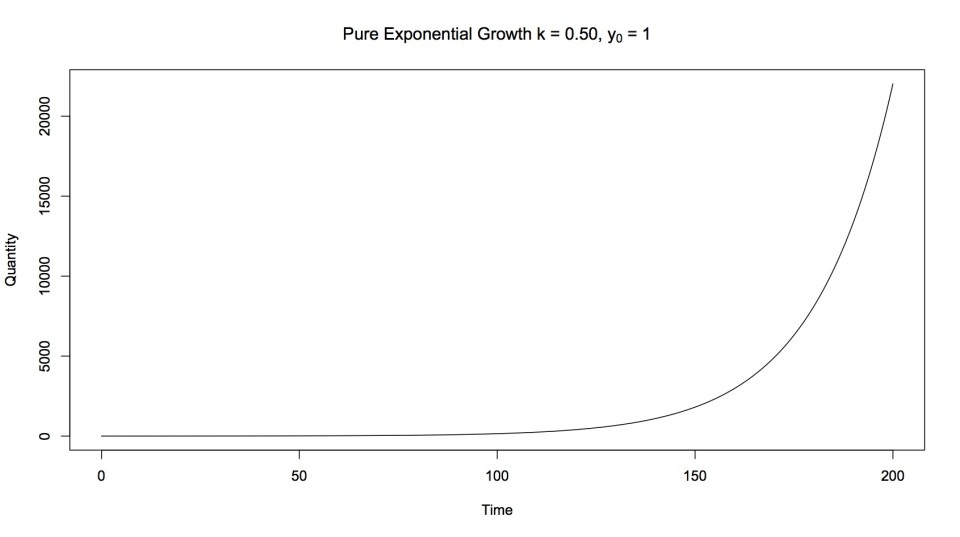# Graphing with R

#### By Mark Ciotola

First published on October 10, 2019. Last updated on February 6, 2021.

## What is R?

“R is a language and environment for for statistical computing and graphics.” In other words, R is primarily intended to perform statistical analysis. However, excellent plots can also be created in R.

R provides both a command line interface, as well as a graphical user interface (GUI). Admittedly, the GUI looks like a command line interface, but it contains several buttons that can save time.R environment window

## Running R

A quick way to learn a few R basics is to create a simple plot.

To start with R, it is easiest to run it from the environment. Under the File menu (at the top of the screen), choose New Document. Save the document as R_simple_plot.

Add the following line at the “>” prompt. This will provide data for a plot. First we state the variable “population” to hold the data. We use the “<-” notation to assign data to that variable. “c()” is a function that you can use to provide data.

population <- c(1000, 1200, 1400, 1500 , 1550)

Then add the below line to tell R to create a plot of the data. Plot is a function. It processes information supplied to it between the parentheses. “Type” describes the format of the plot. “Col” specifies a color for the plot.

plot(population, type = "o", col="blue")

Save the file again. To view the plot, choose the “Source Script” button (the R circle button), then choose your file. You should see a plot similar to that below.Result of R plot

## A More Complicated Example

Below is a more complicated example. You can copy and paste it into a new file to see the result.

x <- 0:200
y <- exp( 0.05 * x )

par(mfrow=c(1,1))

plot(x, y, main=expression(paste("Pure Exponential Growth k = 0.50, ", y, " = 1")), xlab="Time", ylab="Quantity", type = "l")

#abline(lm(y~x))

#abline(lowess(x,y))

#lines(lowess(x,y))

#lines(x, y, type="l")

#title("Power Progression of Romanov Dynasty versus Year")